# How to Calculate Remaining Days in a Month or Year in Excel

This post will guide you how to calculate remaining days in a given month or year in Excel. How do I calculate the number of days left in a month or year using a formula in Excel 2013/2016.

## Calculate Remaining Days in a Month

Assuming that you have a list of data in range B1:B4, in which contain date values, and you want to calculate the remaining days in the current month or year, how to accomplish it. You can use a formula based on the EOMONTH function. like this:

=EOMONTH(B1,0)-B1

Type this formula into a blank cell and press Enter key on your keyboard, and you drag the AutoFill Handle down to ther cells to apply this formula.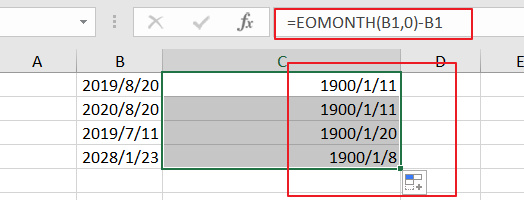And keep to select the result, and then right click on the selected cells, and select Format Cells from the context menu list, and click Number tab, select General category from the left Category list, and click Ok button. You would see that the remaining days in this month is calculated.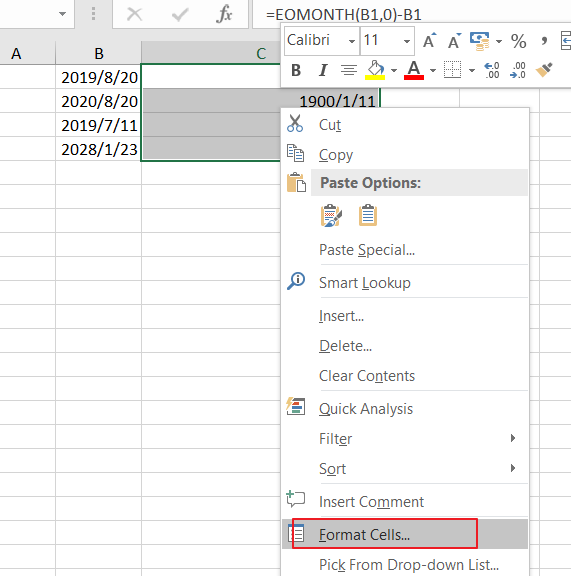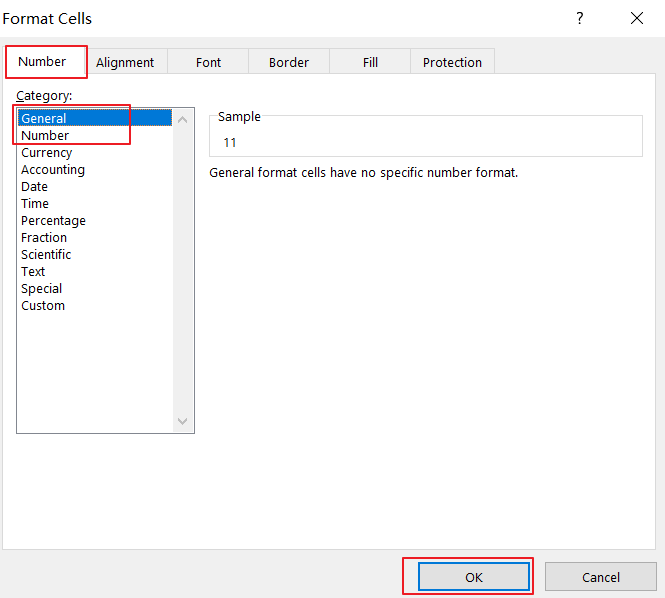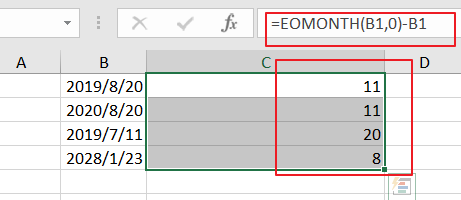## Calculate Remaining Days in a Year

If you want to calculate the remaining days in the given year, you can use another formula based on the DATE function, and the Year function. like this:

=DATE(YEAR(A2),12,31)-A2

Type this formula into a blank cell and press Enter key on your keyboard, and drag the Autofill Handle over to other cells.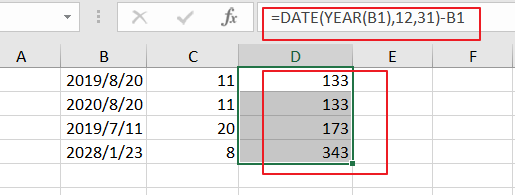### Related Functions

• Excel DATE function
The Excel DATE function returns the serial number for a date.The syntax of the DATE function is as below:= DATE (year, month, day)…
• Excel YEAR function
The Excel YEAR function returns a four-digit year from a given date value, the year is returned as an integer ranging from 1900 to 9999. The syntax of the YEAR function is as below:=YEAR (serial_number)…